# Mapping in Python with geopandas¶

In :
%matplotlib inline

import matplotlib.pyplot as plt
import geopandas as gpd
import pysal as ps
from pysal.contrib.viz import mapping as maps


In this lab, we will learn how to load, manipulate and visualize spatial data. In some senses, spatial data have become so pervasive that nowadays, they are usually included simply as "one more column" in a table. However, spatial is special and there are few aspects in which geographic data differ from standard numerical tables. In this session, we will extend the skills developed in the previous one about non-spatial data, and combine them. In the process, we will discover that, although with some particularities, dealing with spatial data in Python largely resembles dealing with non-spatial data. For example, in this lab you will learn to make slick maps like this one with just a few commands: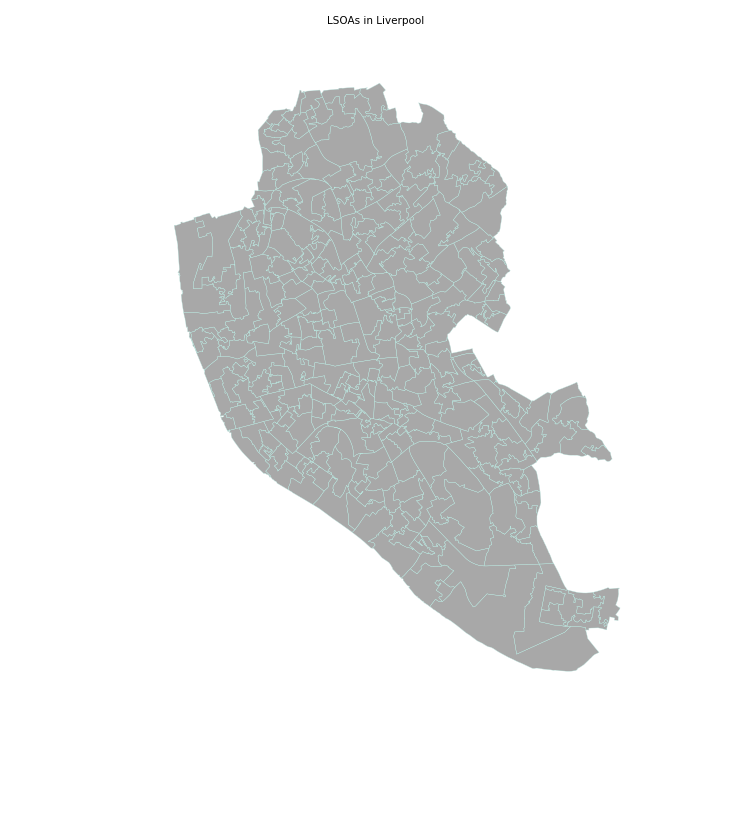Or interactive ones like this one where the smallest areas of Liverpool are highlighted in red:

In :
# Please note this will not display on your machine as it depends on an external file
# A rendered version can be found on the HTML or PDF versions of this notebook
from IPython.display import IFrame
IFrame("figs/lab03_liverpool_smallest.html", width=560, height=315)

Out:

To learn these concepts, we will be playing again with the geography of Liverpool. In particular we will use Census geographies (Available as part of the Census Data pack used before, see link) and Ordnance Survey geospatial data, available to download also from the CDRC data store (link). To make the rest of the notebook easier to follow, let us set the paths to the main two folders here. We will call the path to the Liverpool Census pack lcp_dir, and that to the OS geodata los_dir:

In :
lcp_dir = '../../../../data/Liverpool/'
los_dir = '../../../../data/E08000012_OS/'


The easiest way to get from a file to a quick visualization of the data is by loading it as a GeoDataFrame and calling the plot command. The main library employed for all of this is geopandas which is a geospatial extension of the pandas library, already introduced before. geopandas supports exactly the same functionality that pandas does (in fact since it is built on top of it, so most of the underlying machinery is pure pandas), plus a wide range of spatial counterparts that make manipulation and general "munging" of spatial data as easy as non-spatial tables.

In two lines of code, we will obtain a graphical representation of the spatial data contained in a file that can be in many formats; actually, since it uses the same drivers under the hood, you can load pretty much the same kind of vector files that QGIS permits. Let us start by plotting single layers in a crude but quick form, and we will build style and sophistication into our plots later on.

• Polygons

Let us begin with the most common type of spatial data in the social science: polygons. For example, we can load the geography of LSOAs in Liverpool with the following lines of code:

In :
lsoas_link = lcp_dir + 'shapefiles/Liverpool_lsoa11.shp'


Now lsoas is a GeoDataFrame. Very similar to a traditional, non-spatial DataFrame, but with an additional column called geometry:

In :
lsoas.head()

Out:
LSOA11CD geometry
0 E01006512 POLYGON ((336103.358 389628.58, 336103.416 389...
1 E01006513 POLYGON ((335173.781 389691.538, 335169.798 38...
2 E01006514 POLYGON ((335495.676 389697.267, 335495.444 38...
3 E01006515 POLYGON ((334953.001 389029, 334951 389035, 33...
4 E01006518 POLYGON ((335354.015 388601.947, 335354 388602...

This allows us to quickly produce a plot by executing the following line:

In :
lsoas.plot()

Out:
<matplotlib.axes._subplots.AxesSubplot at 0x10e05f890>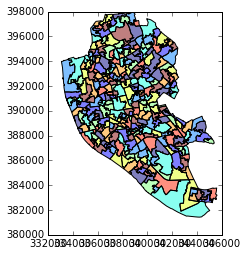This might not be the most aesthetically pleasant visual representation of the LSOAs geography, but it is hard to argue it is not quick to produce. We will work on styling and customizing spatial plots later on.

Pro-tip: if you call a single row of the geometry column, it'll return a small plot ith the shape:

In :
lsoas.loc[0, 'geometry']

Out:
• Lines

Displaying lines is as straight-forward as polygons. To load railway tunnels in Liverpool and name the rows after the id column (or to "index" them):

In :
rwy_tun = gpd.read_file(los_dir + 'RailwayTunnel.shp')
rwy_tun = rwy_tun.set_index('id')
rwy_tun.info()

<class 'geopandas.geodataframe.GeoDataFrame'>
Index: 44 entries, 0ACD196C321E4F8DE050A00A568A6F6F to 0ACD196C313D4F8DE050A00A568A6F6F
Data columns (total 2 columns):
featcode    44 non-null float64
geometry    44 non-null object
dtypes: float64(1), object(1)
memory usage: 1.0+ KB


Note how, similarly to the polygon case, if we pick the "geometry" column of a table with lines, a single row will display the geometry as well:

In :
rwy_tun.loc['0ACD196C313D4F8DE050A00A568A6F6F', 'geometry']

Out:

Note how we have also indexed the table on the id column.

A quick plot is similarly generated by (mind that because there are over 18,000 segments, this may take a little bit):

In :
rwy_tun.plot()

Out:
<matplotlib.axes._subplots.AxesSubplot at 0x110b12f10>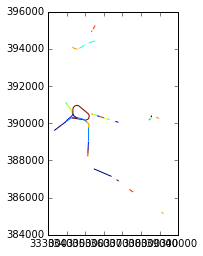Again, this is not the prettiest way to display the roads maybe, and you might want to change a few parameters such as colors, etc. All of this is possible, as we will see below, but this gives us an easy check of what lines look like.

[Optional exercise]

Obtain the graphical representation of the line with id = 0ACD196C32214F8DE050A00A568A6F6F.

• Points

Finally, points follow a similar structure. If we want to represent named places in Liverpool:

In :
namp = gpd.read_file(los_dir + 'NamedPlace.shp')

Out:
classifica distname featcode fontheight geometry htmlname id orientatio
0 Hydrography Sugar Brook 15804 Small POINT (339605 396261) Sugar Brook 0EE7A103C03A8FBFE050A00A568A2502 25
1 Landcover Sandfield Park 15805 Small POINT (339758 392357) Sandfield Park 0EE7A104A4B68FBFE050A00A568A2502 0
2 Populated Place Sandfield Park 15801 Medium POINT (339768 392217) Sandfield Park 0EE7A1041DB18FBFE050A00A568A2502 0
3 Populated Place Gillmoss 15801 Medium POINT (340269 396567) Gillmoss 0EE7A1041DE48FBFE050A00A568A2502 0
4 Populated Place Croxteth 15801 Medium POINT (340296 395304) Croxteth 0EE7A1041DE58FBFE050A00A568A2502 0

And the plot is produced by running:

In :
namp.plot()

Out:
<matplotlib.axes._subplots.AxesSubplot at 0x11124c710>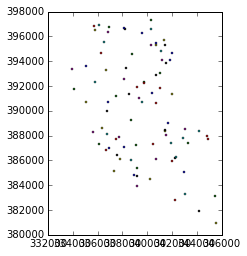## Styling plots¶

It is possible to tweak several aspects of a plot to customize if to particular needs. In this section, we will explore some of the basic elements that will allow us to obtain more compelling maps.

NOTE: some of these variations are very straightforward while others are more intricate and require tinkering with the internal parts of a plot. They are not necessarily organized by increasing level of complexity.

• Changing transparency

The intensity of color of a polygon can be easily changed through the alpha attribute in plot. This is specified as a value betwee zero and one, where the former is entirely transparent while the latter is the fully opaque (maximum intensity):

In :
lsoas.plot(alpha=0.1)

Out:
<matplotlib.axes._subplots.AxesSubplot at 0x111808a50>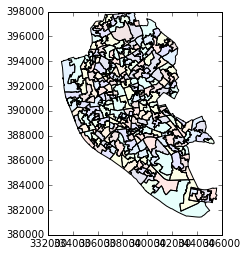In :
lsoas.plot(alpha=1)

Out:
<matplotlib.axes._subplots.AxesSubplot at 0x112750a90>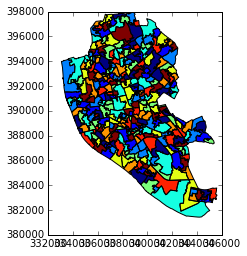• Removing axes

Although in some cases, the axes can be useful to obtain context, most of the times maps look and feel better without them. Removing the axes involves wrapping the plot into a figure, which takes a few more lines of aparently useless code but that, in time, it will allow you to tweak the map further and to create much more flexible designs:

In :
f, ax = plt.subplots(1)
ax = lsoas.plot(axes=ax)
ax.set_axis_off()
plt.show()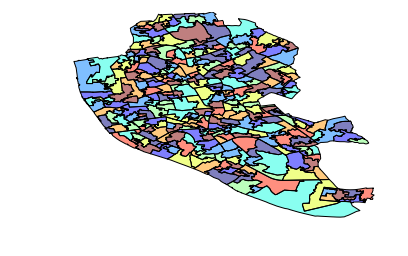Let us stop for a second a study each of the previous lines:

1. We have first created a figure named f with one axis named ax by using the command plt.subplots (part of the library matplotlib, which we have imported at the top of the notebook). Note how the method is returning two elements and we can assign each of them to objects with different name (f and ax) by simply listing them at the front of the line, separated by commas.
2. Second, we plot the geographies as before, but this time we tell the function that we want it to draw the polygons on the axis we are passing, ax. This method returns the axis with the geographies in them, so we make sure to store it on an object with the same name, ax.
3. On the third line, we effectively remove the box with coordinates.
4. Finally, we draw the entire plot by calling plt.show().

Adding a title is a simple extra line, if we are creating the plot within a figure, as we just did. To include text on top of the figure:

In :
f, ax = plt.subplots(1)
ax = lsoas.plot(axes=ax)
f.suptitle('Liverpool LSOAs')
plt.show()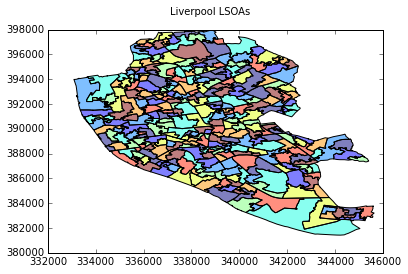• Changing the size of the map

The size of the plot is changed equally easily in this context. The only difference is that it is specified when we create the figure with the argument figsize. The first number represents the width, the X axis, and the second corresponds with the height, the Y axis.

In :
f, ax = plt.subplots(1, figsize=(12, 12))
ax = lsoas.plot(axes=ax)
plt.show()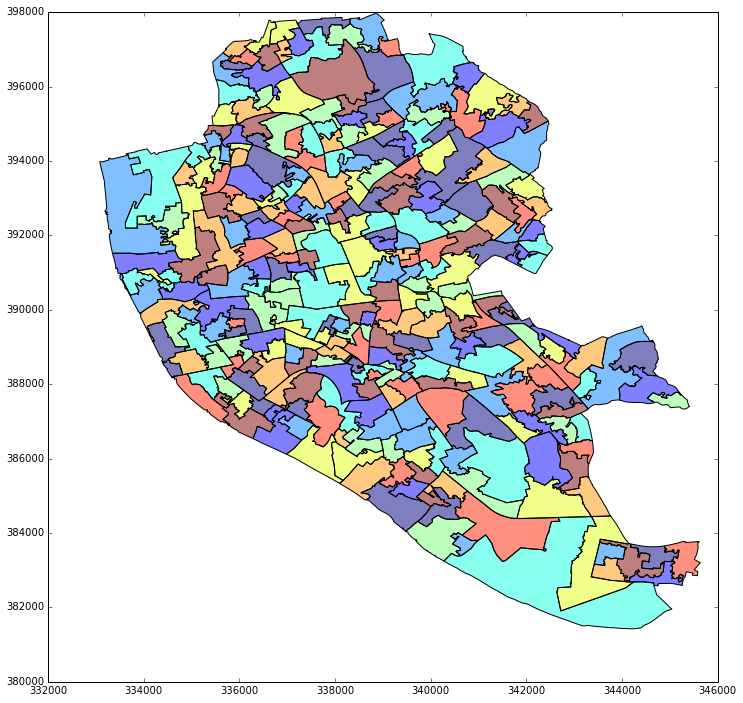• Scaling plots

You will notice that the ability to change the size of the figure is very powerful as it makes possible to obtain many different sizes and shapes for plots. However, this also may introduce some distortions in the way the shapes are represented. For example, a very wide figure can make the viewer think that polygons are in reality more "stretched out" than they are in reality:

In :
f, ax = plt.subplots(1, figsize=(12, 4))
ax = lsoas.plot(axes=ax)
plt.show()